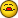# Efficiency of Code Help

J

#### Jbm

Hey,
If you want to get right to the code, it's at the bottom, but here's an
explanation of what it's meant to do first if you'd like.

I'm certain there's redundancy in the code, and because of this it's fairly
slow for the relatively simple function it's performing. Essentially, I have
a new workbook produced every day that has data for that entire month, up to
and including that day. This runs from Column A to E, and anywhere from
about 200 to 7000 rows. Column A consists of dates, B of just words, and C-E
are numerical. The task is, on the same sheet, to show all data pertaining
to only 6 keywords for only the previous date. So if it's the 29th today, I
receive a worksheet, and data from the 28th that has one of the six keywords
needs to go in columns G-K. Since this happens everyday, copying/pasting a
macro off a word document and hitting run seems to be the fastest rather than
doing it manually or having to change the code every time.

If you can improve the running time of this code while retaining the same
end-output, it would be greatly appreciated. Thanks to any and all.

Sub DataMove()
RowCount = 1
For Each c In Range("B:B")
If c.Value Like "*Ask Jeeves organic*" Or _
c.Value Like "*Unified Sources (evar17)*" Or _
c.Value Like "*Google organic*" Or _
c.Value Like "*Microsoft Bing organic*" Or _
c.Value Like "*Live.com organic*" Or _
c.Value Like "*Yahoo! organic*" Or _
c.Value Like "*AOL.com Search organic*" Then
Cells(RowCount, "S").Value = c.Value
Cells(RowCount, "T").Value = c.Offset(0, 1).Value
Cells(RowCount, "U").Value = c.Offset(0, 2).Value
Cells(RowCount, "V").Value = c.Offset(0, 3).Value
Cells(RowCount, "R").Value = c.Offset(0, -1).Value
RowCount = RowCount + 1
End If
Next
Range("P1").Value = (Range("A" & Rows.Count).End(xlUp)) - 1
RowCount = 1
For Each c In Range("R:R")
If c.Value = Sheet1.Range("P1").Value Or _
c.Value = "Date" Then
Cells(RowCount, "G").Value = c.Value
Cells(RowCount, "H").Value = c.Offset(0, 1).Value
Cells(RowCount, "I").Value = c.Offset(0, 2).Value
Cells(RowCount, "J").Value = c.Offset(0, 3).Value
Cells(RowCount, "K").Value = c.Offset(0, 4).Value
RowCount = RowCount + 1
End If
Next
Range("R1:V500").Delete
Range(â€œP11â€).Delete
ActiveSheet.UsedRange.AutoFormat
End Sub

J

#### jasontferrell

It's difficult to test without data, but here's an attempt.

Sub DataMove()
RowCount = 1
With ActiveSheet
For RowCount = 1 To .UsedRange.Rows.Count
If .Cells(RowCount, 2).Value Like "*Ask Jeeves organic*" Or _
.Cells(RowCount, 2).Value Like "*Unified Sources
(evar17)*" Or _
.Cells(RowCount, 2).Value Like "*Google organic*" Or _
.Cells(RowCount, 2).Value Like "*Microsoft Bing
organic*" Or _
.Cells(RowCount, 2).Value Like "*Live.com organic*" Or
_
.Cells(RowCount, 2).Value Like "*Yahoo! organic*" Or _
.Cells(RowCount, 2).Value Like "*AOL.com Search
organic*" Then
.Cells(RowCount, 19).Value = .Cells(RowCount, 2).Value
.Cells(RowCount, 20).Value = .Cells(RowCount, 2).Offset(0,
1).Value
.Cells(RowCount, 21).Value = .Cells(RowCount, 2).Offset(0,
2).Value
.Cells(RowCount, 22).Value = .Cells(RowCount, 2).Offset(0,
3).Value
.Cells(RowCount, 18).Value = .Cells(RowCount, 2).Offset(0,
-1).Value
End If
If .Cells(RowCount, 18).Value = (.Range("A" & Rows.Count).End
(xlUp)) - 1 Or _
.Cells(RowCount, 18).Value = "Date" Then
.Cells(RowCount, 7).Value = .Cells(RowCount, 18).Value
.Cells(RowCount, 8).Value = .Cells(RowCount, 18).Offset(0,
1).Value
.Cells(RowCount, 9).Value = .Cells(RowCount, 18).Offset(0,
2).Value
.Cells(RowCount, 10).Value = .Cells(RowCount, 18).Offset
(0, 3).Value
.Cells(RowCount, 11).Value = .Cells(RowCount, 18).Offset
(0, 4).Value
End If
Next RowCount
.Range("P1").Value = (.Range("A" & Rows.Count).End(xlUp)) - 1
.Range("R1:V500").Delete
.Range("P11").Delete
.UsedRange.AutoFormat
End With
End Sub

D

#### Don Guillett

If there is only ONE instance of each text to look for it should be more
efficient to use vba FIND instead. If more, use FINDNEXT

Sub findem()
For Each i In Array("a", "b", "c")'use your text

Set found = Columns("B").Find(What:=i, _
After:=Cells(1, 2), LookIn:=xlValues, _
LookAt:=xlWhole, SearchOrder:=xlByRows, _
SearchDirection:=xlNext, _
MatchCase:=False)

Cells(found.row, "S").Value = cells(found.row,2).Value
Cells(found.Row, 3) = "x"
'etc

End If
Next i
End Sub

K

#### KC

I assume the data is stacked with latest date at the bottom of Col A.

I would
get the latest date;
find the beginning row of previous date ;
find the last row of previous date;
find the keywords within this block;
the data required is contiguous, copy row by row.

D

#### Don Guillett

If desired, send your file to my address below along with this msg and
a clear explanation of what you want and before/after examples.